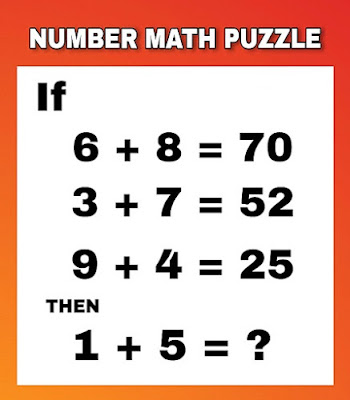# GkJobs

GkJobs

## Number Math Puzzle With Answer | Cool Math Puzzle | Gkjobs.in

Number Math Puzzle With Answer | Cool Math Puzzle | Gkjobs.in# Can you solve this high IQ number math puzzle?

If
6 + 8 = 70
3 + 7 = 52
9 + 4 = 25
Then
1 + 5 = ?
So were you able to guess the answer? if yes then congratulation if no don't worry scroll down further to know the right answer.

EXPLANATION-:

EQUATION 1 -: 6 + ( 8 x 8 ) = 70

EQUATION 2 -: 3 + ( 7 x 7 ) = 52

EQUATION 3 -: 9 + ( 4 x 4 ) = 25

EQUATION 4 -: 1 + ( 5 x 5 ) = 26

THANKS FOR VISITING THIS WEBSITE
HAVE A GOOD DAY.

Tag : Online Test